### 介绍### 目录

1. 迁移学习概论

1. 什幺是预训练模型?如何选择正确的预训练模型?

1. 案例研究:紧急与非紧急车辆分类

1. 使用卷积神经网络(CNNs)解决挑战

1. 使用PyTorch的迁移学习解决挑战

1. CNN的性能比较和迁移学习

### 迁移学习概论### 什幺是预训练模型?如何选择正确的预训练模型?#### ImageNet与MNIST### 案例研究:紧急与非紧急车辆分类csv文件有两列:

1. image_names:它表示数据集中所有图像的名称

1. emergency

or

1. no:它指定特定的图像属于紧急类还是非紧急类。0表示图像是非紧急车辆，1表示紧急车辆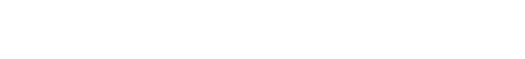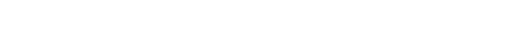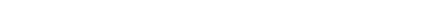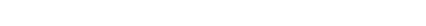### 使用卷积神经网络(CNNs)解决挑战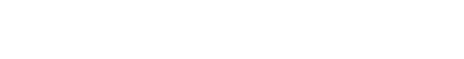```# 验证集预测
prediction_val = []
target_val = []
permutation = torch.randperm(val_x.size())
for i in tqdm(range(0,val_x.size(), batch_size)):
indices = permutation[i:i+batch_size]
batch_x, batch_y = val_x[indices], val_y[indices]
if torch.cuda.is_available():
batch_x, batch_y = batch_x.cuda(), batch_y.cuda()
output = model(batch_x.cuda())
softmax = torch.exp(output).cpu()
prob = list(softmax.numpy())
predictions = np.argmax(prob, axis=1)
prediction_val.append(predictions)
target_val.append(batch_y)

# 验证集精确度
accuracy_val = []
for i in range(len(prediction_val)):
accuracy_val.append(accuracy_score(target_val[i],prediction_val[i]))

print('validation accuracy: \t', np.average(accuracy_val))```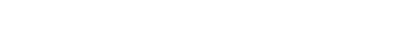### 使用PyTorch的迁移学习解决挑战

1. 首先，我们将加载预训练模型的权重——在我们的例子中是VGG16

1. 然后我们将根据手头的问题对模型进行微调

1. 接下来，我们将使用这些预训练的权重并提取图像的特征

1. 最后，我们将使用提取的特征训练精细调整的模型

```# 加载预训练模型
model = models.vgg16_bn(pretrained=True)```

```# 固定模型权重
for param in model.parameters():

```# 最后加一个分类器
model.classifier = Sequential(
Linear(4096, 2))
for param in model.classifier.parameters():

```# 检查GPU是否可用
if torch.cuda.is_available():
model = model.cuda()```

```# batch大小
batch_size = 128
# 从训练集提取特征
data_x = []
label_x = []
inputs,labels = train_x, train_y
for i in tqdm(range(int(train_x.shape/batch_size)+1)):
input_data = inputs[i*batch_size:(i+1)*batch_size]
label_data = labels[i*batch_size:(i+1)*batch_size]
input_data , label_data = Variable(input_data.cuda()),Variable(label_data.cuda())
x = model.features(input_data)
data_x.extend(x.data.cpu().numpy())
label_x.extend(label_data.data.cpu().numpy())```

```# 从验证集提取特征
data_y = []
label_y = []
inputs,labels = val_x, val_y
for i in tqdm(range(int(val_x.shape/batch_size)+1)):
input_data = inputs[i*batch_size:(i+1)*batch_size]
label_data = labels[i*batch_size:(i+1)*batch_size]
input_data , label_data = Variable(input_data.cuda()),Variable(label_data.cuda())
x = model.features(input_data)
data_y.extend(x.data.cpu().numpy())
label_y.extend(label_data.data.cpu().numpy())```

```# 转换这些数据到torch格式
x_train  = torch.from_numpy(np.array(data_x))
x_train = x_train.view(x_train.size(0), -1)
y_train  = torch.from_numpy(np.array(label_x))
x_val  = torch.from_numpy(np.array(data_y))
x_val = x_val.view(x_val.size(0), -1)
y_val  = torch.from_numpy(np.array(label_y))```

```# batch大小
batch_size = 128
# 30个epochs
n_epochs = 30
for epoch in tqdm(range(1, n_epochs+1)):
# 跟踪训练与验证集损失
train_loss = 0.0

permutation = torch.randperm(x_train.size())
training_loss = []
for i in range(0,x_train.size(), batch_size):
indices = permutation[i:i+batch_size]
batch_x, batch_y = x_train[indices], y_train[indices]

if torch.cuda.is_available():
batch_x, batch_y = batch_x.cuda(), batch_y.cuda()

outputs = model.classifier(batch_x)
loss = criterion(outputs,batch_y)
training_loss.append(loss.item())
loss.backward()
optimizer.step()

training_loss = np.average(training_loss)
print('epoch: \t', epoch, '\t training loss: \t', training_loss)``````# 预测训练集
prediction = []
target = []
permutation = torch.randperm(x_train.size())
for i in tqdm(range(0,x_train.size(), batch_size)):
indices = permutation[i:i+batch_size]
batch_x, batch_y = x_train[indices], y_train[indices]
if torch.cuda.is_available():
batch_x, batch_y = batch_x.cuda(), batch_y.cuda()
output = model.classifier(batch_x.cuda())
softmax = torch.exp(output).cpu()
prob = list(softmax.numpy())
predictions = np.argmax(prob, axis=1)
prediction.append(predictions)
target.append(batch_y)

# 训练精度
accuracy = []
for i in range(len(prediction)):
accuracy.append(accuracy_score(target[i],prediction[i]))

print('training accuracy: \t', np.average(accuracy))```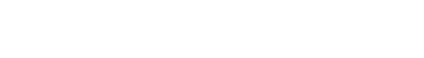```# 预测验证集
prediction = []
target = []
permutation = torch.randperm(x_train.size())
for i in tqdm(range(0,x_train.size(), batch_size)):
indices = permutation[i:i+batch_size]
batch_x, batch_y = x_train[indices], y_train[indices]
if torch.cuda.is_available():
batch_x, batch_y = batch_x.cuda(), batch_y.cuda()
output = model.classifier(batch_x.cuda())
softmax = torch.exp(output).cpu()
prob = list(softmax.numpy())
predictions = np.argmax(prob, axis=1)
prediction.append(predictions)
target.append(batch_y)

# 验证精度
accuracy = []
for i in range(len(prediction)):
accuracy.append(accuracy_score(target[i],prediction[i]))

print('training accuracy: \t', np.average(accuracy))```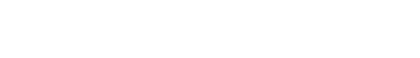Model Training Accuracy Validation Accuracy
CNN 81.57% 76.26%
83.70% 83.47%